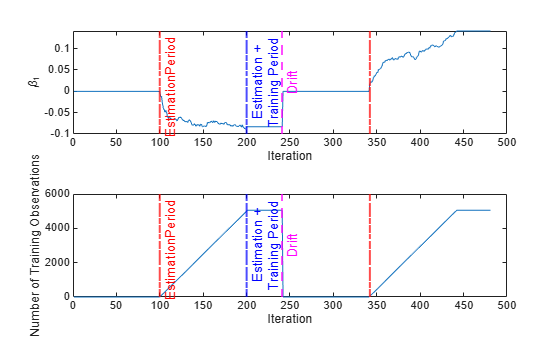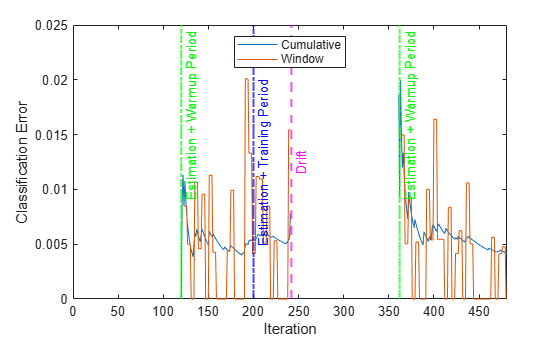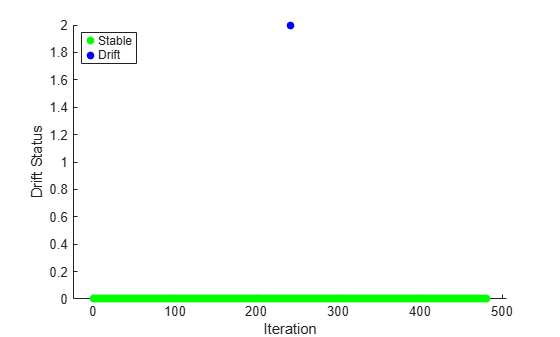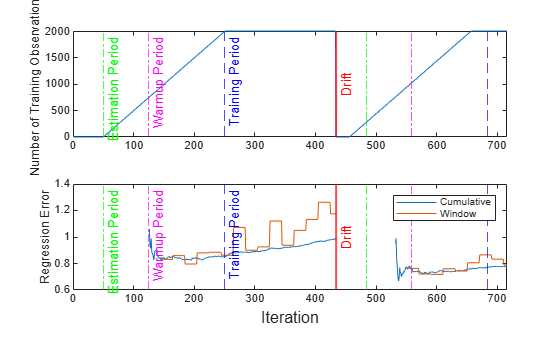# fit

Train drift-aware learner for incremental learning with new data

Since R2022b

## Syntax

Mdl = fit(Mdl,X,Y)
Mdl = fit(Mdl,X,Y,Name=Value)

## Description

example

Mdl = fit(Mdl,X,Y) returns an incremental drift-aware learning model Mdl, which represents the input incremental drift-aware learning model Mdl trained using the predictor and response data, X and Y, respectively.fit does not update Mdl.Metrics.

example

Mdl = fit(Mdl,X,Y,Name=Value) uses additional options specified by one or more name-value arguments. For example, you can specify that the columns of the predictor data matrix correspond to observations, and set observation weights.

## Examples

collapse all

Load the human activity dataset. Randomly shuffle the data.

load humanactivity; n = numel(actid); rng(1) % For reproducibility idx = randsample(n,n);

For details on the data set, enter Description at the command line.

Define the predictor and response variables.

X = feat(idx,:); Y = actid(idx);

Responses can be one of five classes: Sitting, Standing, Walking, Running, or Dancing.

Dichotomize the response by identifying whether the subject is moving (actid > 2).

Y = Y > 2;

Flip labels for the second half of the dataset to simulate drift.

Y(floor(numel(Y)/2):end,:) = ~Y(floor(numel(Y)/2):end,:);

Initiate a default incremental drift-aware model for classification as follows:

1. Create an incremental linear SVM model for binary classification. Specify an estimation period of 5000 observations and the SGD solver.

2. Initiate a default incremental drift-aware model using the incremental linear SVM model as the base learner.

baseMdl = incrementalClassificationLinear(EstimationPeriod=5000,Solver="sgd"); idaMdl = incrementalDriftAwareLearner(baseMdl);

idaMdl is an incrementalDriftAwareLearner model. All its properties are read-only. By default, incrementalDriftAwareLearner uses the Hoeffding's Bound drift detection method based on moving averages ("hddma").

idaMdl must be fit to data before you can use it to perform any other operations.

Fit the incremental drift-aware model to the training data, in chunks of 50 observations at a time, by using the fit function. At each iteration:

1. Simulate a data stream by processing 50 observations.

2. Overwrite the previous incremental model with a new one fitted to the incoming observations.

3. Store the number of training observations, and the prior probability of whether the subject moved (Y = true) to see how they evolve during incremental training.

% Preallocation numObsPerChunk = 50; nchunk = floor(n/numObsPerChunk); beta1 = zeros(nchunk,1); numtrainobs = zeros(nchunk,1); dstatus = zeros(nchunk,1); statusname = strings(nchunk,1); driftTimes = []; ce = array2table(zeros(nchunk,2),VariableNames=["Cumulative" "Window"]); % Incremental fitting for j = 1:nchunk ibegin = min(n,numObsPerChunk*(j-1) + 1); iend = min(n,numObsPerChunk*j); idx = ibegin:iend; idaMdl = fit(idaMdl,X(idx,:),Y(idx)); idaMdl = updateMetrics(idaMdl,X(idx,:),Y(idx)); beta1(j) = idaMdl.BaseLearner.Beta(1); % Record drift status and classification error statusname(j) = string(idaMdl.DriftStatus); ce{j,:} = idaMdl.Metrics{"ClassificationError",:}; numtrainobs(j) = idaMdl.NumTrainingObservations; if idaMdl.DriftDetected dstatus(j) = 2; driftTimes(end+1) = j; elseif idaMdl.WarningDetected dstatus(j) = 1; else dstatus(j) = 0; end end

idaMdl is an incrementalDriftAwareLearner model object trained on all the data in the stream.

To see how the parameters evolve during incremental learning, plot them on separate tiles.

tiledlayout(2,1) nexttile plot(beta1) ylabel("\beta_1") xline(idaMdl.BaseLearner.EstimationPeriod/numObsPerChunk,"r-.","EstimationPeriod") xline(idaMdl.BaseLearner.EstimationPeriod/numObsPerChunk + driftTimes,"r-.") xlabel('Iteration') xline(idaMdl.TrainingPeriod/numObsPerChunk,"b-.","Training Period",LabelVerticalAlignment="middle",LineWidth= 1.5) xline(floor(numel(Y)/2)/numObsPerChunk,"m--","Drift",LabelVerticalAlignment="middle",LineWidth= 1.5) axis tight nexttile plot(numtrainobs) ylabel("Number of Training Observations") xline(idaMdl.BaseLearner.EstimationPeriod/numObsPerChunk,"r-.","EstimationPeriod") xline(idaMdl.BaseLearner.EstimationPeriod/numObsPerChunk + driftTimes,"r-.") xlabel("Iteration") xline(idaMdl.TrainingPeriod/numObsPerChunk,"b-.","Training Period",LabelVerticalAlignment="middle") xline(floor(numel(Y)/2)/numObsPerChunk,"m--","Drift",LabelVerticalAlignment="middle") axis tightThe plot suggests that fit does not fit the model to the data or update the parameters until after the estimation period. After a drift is detected, the function waits for another Mdl.BaseLearner.EstimationPeriod number of observations to fit the new model to data.

Plot the cumulative and per window classification error. Mark the warmup and training periods, and where the drift was introduced.

figure() h = plot(ce.Variables); xlim([0 nchunk]) ylabel("Classification Error") xlabel("Iteration") xline((idaMdl.BaseLearner.EstimationPeriod+idaMdl.MetricsWarmupPeriod)/numObsPerChunk,"g-.","Estimation + Warmup Period",LineWidth=1.5) xline((idaMdl.BaseLearner.EstimationPeriod+idaMdl.MetricsWarmupPeriod)/numObsPerChunk+driftTimes,"g-.","Estimation + Warmup Period",LineWidth=1.5) xline(idaMdl.TrainingPeriod/numObsPerChunk,"b-.","Training Period",LabelVerticalAlignment="middle",LineWidth=1.5) xline(driftTimes,"m--","Drift",LabelVerticalAlignment="middle",LineWidth=1.5) legend(h,ce.Properties.VariableNames) legend(h,Location="best")Plot the drift status versus the iteration number.

gscatter(1:nchunk,dstatus,statusname,"gbr","o",5,"on","Iteration","Drift Status","filled")Predict labels for the second half of the data and check the accuracy of the model updated after the drift.

n = floor(numel(Y)/2); yhat = predict(idaMdl,X(n:end,:)); accuracy = sum(Y(n:end)==yhat)/n
accuracy = 0.9903 

Load the robotarm data set. Obtain the sample size n and the number of predictor variables p.

load robotarm n = numel(ytrain); p = size(Xtrain,2);

For details on the data set, enter Description at the command line.

Introduce an artificial drift to the response variable between observations 2500 and 5000.

Y=ytrain; j=1.25; for i=2500:1250:5000 idx=min(i+1250,5000); Y(i:idx)=ytrain(i:idx)*j; j=j+0.25; end

Initiate an incremental drift-aware model for regression as follows:

1. Create an incremental linear SVM model for regression. Specify an estimation period of 500 observations and the SGD solver.

2. Create an incremental drift detector for continuous data.

3. Initiate an incremental drift-aware model using the incremental linear SVM model as the base learner and the drift detector you created. Specify a training period of 2000.

baseMdl = incrementalRegressionLinear(EstimationPeriod=500,Solver="sgd",MetricsWarmUpPeriod=750); ddetector = incrementalConceptDriftDetector("hddma",InputType="continuous",Alternative="greater"); idaMdl = incrementalDriftAwareLearner(baseMdl,DriftDetector=ddetector,TrainingPeriod=2000);

idaMdl is an incrementalDriftAwareLearner model. All its properties are read-only.

Preallocate the number of variables in each chunk and number of iterations for creating a stream of data.

numObsPerChunk = 10; nchunk = floor(n/numObsPerChunk);

Preallocate the variables for tracking the drift status and drift time, and storing the regression error and number of training observations.

dstatus = zeros(nchunk,1); statusname = strings(nchunk,1); driftTimes = []; ei = array2table(nan(nchunk,2),VariableNames=["Cumulative","Window"]); numtrainobs = zeros(nchunk,1);

Perform incremental learning on the rest of the data by using the updateMetrics and fit functions. At each iteration:

1. Simulate a data stream by processing 10 observations at a time.

2. Call updateMetrics to update the cumulative and window classification error of the model given the incoming chunk of observations. Overwrite the previous incremental model to update the losses in the Metrics property. Note that the function does not fit the model to the chunk of new data. Specify the observation orientation.

3. Call fit to fit the model to the incoming chunk of observations. Overwrite the previous incremental model to update the model parameters. Specify the observation orientation.

4. Store the regression error and number of training observations.

rng(123) % For reproducibility for j = 1:nchunk ibegin = min(n,numObsPerChunk*(j-1) + 1); iend = min(n,numObsPerChunk*j); idx = ibegin:iend; idaMdl = updateMetrics(idaMdl,Xtrain(idx,:),Y(idx),ObservationsIn="rows"); ei{j,:} = idaMdl.Metrics{"EpsilonInsensitiveLoss",:}; idaMdl = fit(idaMdl,Xtrain(idx,:),Y(idx),ObservationsIn="rows"); numtrainobs(j) = idaMdl.NumTrainingObservations; statusname(j) = string(idaMdl.DriftStatus); if idaMdl.DriftDetected dstatus(j) = 2; driftTimes(end+1) = j; elseif idaMdl.WarningDetected dstatus(j) = 1; else dstatus(j) = 0; end end

idaMdl is an incrementalDriftAwareModel object trained on all the data in the stream.

Plot a trace plot of the number of training observations and the performance metrics. Mark the times for estimation period, warm up metric period, and training period.

t = tiledlayout(2,1); nexttile plot(numtrainobs) xline(idaMdl.BaseLearner.EstimationPeriod/numObsPerChunk,"g-.","Estimation Period") xline((idaMdl.BaseLearner.EstimationPeriod+idaMdl.MetricsWarmupPeriod)/numObsPerChunk,"m-.","Warmup Period") xline((idaMdl.BaseLearner.EstimationPeriod+idaMdl.TrainingPeriod)/numObsPerChunk,"b--","Training Period") xline(idaMdl.BaseLearner.EstimationPeriod/numObsPerChunk+driftTimes,"g-.") xline((idaMdl.BaseLearner.EstimationPeriod+idaMdl.MetricsWarmupPeriod)/numObsPerChunk+driftTimes,"m-.") xline((idaMdl.BaseLearner.EstimationPeriod+idaMdl.TrainingPeriod)/numObsPerChunk+driftTimes,"b--") xline(driftTimes,"r","Drift",LabelVerticalAlignment="middle",LineWidth=1.5) xlim([0 nchunk]) ylabel("Number of Training Observations") nexttile plot(ei.Variables) xline(idaMdl.BaseLearner.EstimationPeriod/numObsPerChunk,"g-.","Estimation Period") xline((idaMdl.MetricsWarmupPeriod+idaMdl.BaseLearner.EstimationPeriod)/numObsPerChunk,"m-.","Warmup Period") xline((idaMdl.BaseLearner.EstimationPeriod+idaMdl.TrainingPeriod)/numObsPerChunk,"b--","Training Period") xline(idaMdl.BaseLearner.EstimationPeriod/numObsPerChunk+driftTimes,"g-.") xline((idaMdl.BaseLearner.EstimationPeriod+idaMdl.MetricsWarmupPeriod)/numObsPerChunk+driftTimes,"m-.") xline((idaMdl.BaseLearner.EstimationPeriod+idaMdl.TrainingPeriod)/numObsPerChunk+driftTimes,"b--") xline(driftTimes,"r","Drift",LabelVerticalAlignment="middle",LineWidth=1.5) xlim([0 nchunk]) legend(ei.Properties.VariableNames,Location="northeast") ylabel("Regression Error") xlabel(t,"Iteration")Plot the drift status versus the iteration number.

figure() gscatter(1:nchunk,dstatus,statusname,'gmr','*',5,'on',"Iteration","Drift Status")## Input Arguments

collapse all

Incremental drift-aware learning model fit to streaming data, specified as an incrementalDriftAwareLearner model object. You can create Mdl using the incrementalDriftAwareLearner function. For more details, see the object reference page.

Chunk of predictor data to which the model is fit, specified as a floating-point matrix of n observations and Mdl.BaseLearner.NumPredictors predictor variables.

When Mdl.BaseLearner accepts the ObservationsIn name-value argument, the value of ObservationsIn determines the orientation of the variables and observations. The default ObservationsIn value is "rows", which indicates that observations in the predictor data are oriented along the rows of X.

The length of the observation responses (or labels) Y and the number of observations in X must be equal; Y(j) is the response (or label) of observation j (row or column) in X.

Note

• If Mdl.BaseLearner.NumPredictors = 0, fit infers the number of predictors from X, and sets the corresponding property of the output model. Otherwise, if the number of predictor variables in the streaming data changes from Mdl.BaseLearner.NumPredictors, fit issues an error.

• fit supports only floating-point input predictor data. If your input data includes categorical data, you must prepare an encoded version of the categorical data. Use dummyvar to convert each categorical variable to a numeric matrix of dummy variables. Then, concatenate all dummy variable matrices and any other numeric predictors. For more details, see Dummy Variables.

Data Types: single | double

Chunk of responses (or labels) to which the model is fit, specified as one of the following:

• Floating-point vector of n elements for regression models, where n is the number of rows in X.

• Categorical, character, or string array, logical vector, or cell array of character vectors for classification models. If Y is a character array, it must have one class label per row. Otherwise, Y must be a vector with n elements.

The length of Y and the number of observations in X must be equal; Y(j) is the response (or label) of observation j (row or column) in X.

For classification problems:

• When Mdl.BaseLearner.ClassNames is nonempty, the following conditions apply:

• If Y contains a label that is not a member of Mdl.BaseLearner.ClassNames, fit issues an error.

• The data type of Y and Mdl.BaseLearner.ClassNames must be the same.

• When Mdl.BaseLearner.ClassNames is empty, fit infers Mdl.BaseLearner.ClassNames from data.

Data Types: single | double | categorical | char | string | logical | cell

### Name-Value Arguments

Specify optional pairs of arguments as Name1=Value1,...,NameN=ValueN, where Name is the argument name and Value is the corresponding value. Name-value arguments must appear after other arguments, but the order of the pairs does not matter.

Example: ObservationsIn="columns",Weights=W specifies that the columns of the predictor matrix correspond to observations, and the vector W contains observation weights to apply during incremental learning.

Predictor data observation dimension, specified as "columns" or "rows".

fit supports ObservationsIn only if Mdl.BaseLearner supports the ObservationsIn name-value argument.

Example: ObservationsIn="columns"

Data Types: char | string

Chunk of observation weights, specified as a floating-point vector of positive values. fit weighs the observations in X with the corresponding values in Weights. The size of Weights must equal n, which is the number of observations in X.

By default, Weights is ones(n,1).

Example: Weights=w

Data Types: double | single

## Output Arguments

collapse all

Updated incremental drift-aware learning model, returned as an incremental drift-aware learning model object of the same data type as the input model Mdl, incrementalDriftAwareLearner.

If Mdl.BaseLearner.EstimationPeriod > 0, the incremental fitting functions updateMetricsAndFit and fit estimate hyperparameters using the first Mdl.BaseLearner.EstimationPeriod observations passed to either function; they do not train the input model to the data. However, if an incoming chunk of n observations is greater than or equal to the number of observations remaining in the estimation period m, fit estimates hyperparameters using the first nm observations, and fits the input model to the remaining m observations.

For classification problems, if the ClassNames property of the input model Mdl.BaseLearner is an empty array, fit sets the ClassNames property of the output model Mdl.BaseLearner to unique(Y).

## Algorithms

collapse all

### Incremental Drift-Aware Learning

Incremental learning, or online learning, is a branch of machine learning concerned with processing incoming data from a data stream, possibly given little to no knowledge of the distribution of the predictor variables, aspects of the prediction or objective function (including tuning parameter values), or whether the observations are labeled. Incremental learning differs from traditional machine learning, where enough labeled data is available to fit to a model, perform cross-validation to tune hyperparameters, and infer the predictor distribution. For more details, see Incremental Learning Overview.

Unlike other incremental learning functionality offered by Statistics and Machine Learning Toolbox™, fit model object combines incremental learning and concept drift detection.

After creating an incrementalDriftAwareLearner object, use updateMetrics to update model performance metrics and fit to fit the base model to incoming chunk of data, check for potential drift in the model performance (concept drift), and update or reset the incremental drift-aware learner, if necessary. You can also use updateMetricsAndFit. The fit function implements the Reactive Drift Detection Method (RDDM)  as follows:

• After Mdl.BaseLearner.EstimationPeriod (if necessary) and MetricsWarmupPeriod, the function trains the incremental drift-aware model up to NumTrainingObservations observations until it reaches TrainingPeriod. (If the TrainingPeriod value is smaller than the Mdl.BaseLearner.MetricsWarmupPeriod value, then incrementalDriftAwareLearner sets the TrainingPeriod value as Mdl.BaseLearner.MetricsWarmupPeriod.)

• When NumTrainingObservations > TrainingPeriod, the software starts tracking the model loss. The software computes the per observation loss using the perObservationLoss function. While computing the per observation loss, the software uses the "classiferror" loss metric for classification models and "squarederror" for regression models. The function then appends the loss values computed using the last chunk of data to the existing buffer loss values.

• Next, the software checks to see if any concept drift occurred by using the detectdrift function and updates DriftStatus accordingly.

Based on the drift status, fit performs the following procedure:

• DriftStatus is 'Warning' The software first increases the consecutive 'Warning' status count by 1.

• If the consecutive 'Warning' status count is less than the WarningCountLimit value and the PreviousDriftStatus value is Stable, then the software trains a temporary incremental learner (if one does not exist) and sets it (or the existing one) to BaseLearner.

Then the software resets the temporary incremental learner using the learner's reset function.

• If the consecutive 'Warning' status count is less than the WarningCountLimit value and the PreviousDriftStatus value is 'Warning', then the software trains the existing temporary incremental model using the latest chunk of data.

• If the consecutive 'Warning' status count is more than the WarningCountLimit value, then the software sets the DriftStatus value to 'Drift'.

• DriftStatus is 'Drift' The software performs the following steps.

• Sets the consecutive 'Warning' status count to 0.

• Resets DriftDetector using the reset function.

• Empties the buffer loss values and appends the loss values for the latest chunk of data to buffer loss values.

• If the temporary incremental model is not empty, then the software sets the current BaseLearner value to the temporary incremental model and empties the temporary incremental model.

• If the temporary incremental model is empty, then the software resets the BaseLearner value by using the learner's reset function.

• DriftStatus is 'Stable' The software first increases the consecutive 'Stable' status count by 1.

• If the consecutive 'Stable' status count is less than the StableCountLimit and the PreviousDriftStatus value is 'Warning', then the software sets the number of warnings to zero and empties the temporary model.

• If the consecutive 'Stable' status count is more than the StableCountLimit value, then the software resets the DriftDetector using the reset function. Then the software tests all of the saved loss values in the buffer for concept drift by using the detectdrift function.

Once DriftStatus is set to 'Drift', and the BaseLearner and DriftDetector are reset, the software waits until Mdl.BaseLearner.EstimationPeriod + Mdl.BaseLearner.MetricsWarmupPeriod before it starts computing the performance metrics.

### Observation Weights

For classification problems, if the prior class probability distribution is known (in other words, the prior distribution is not empirical), fit normalizes observation weights to sum to the prior class probabilities in the respective classes. This action implies that observation weights are the respective prior class probabilities by default.

For regression problems or if the prior class probability distribution is empirical, the software normalizes the specified observation weights to sum to 1 each time you call fit.

 Barros, Roberto S.M. , et al. "RDDM: Reactive drift detection method." Expert Systems with Applications. vol. 90, Dec. 2017, pp. 344-55. https://doi.org/10.1016/j.eswa.2017.08.023.

 Bifet, Albert, et al. "New Ensemble Methods for Evolving Data Streams." Proceedings of the 15th ACM SIGKDD International Conference on Knowledge Discovery and Data Mining. ACM Press, 2009, p. 139. https://doi.org/10.1145/1557019.1557041.

 Gama, João, et al. "Learning with drift detection". Advances in Artificial Intelligence – SBIA 2004, edited by Ana L. C. Bazzan and Sofiane Labidi, vol. 3171, Springer Berlin Heidelberg, 2004, pp. 286–95. https://doi.org/10.1007/978-3-540-28645-5_29.